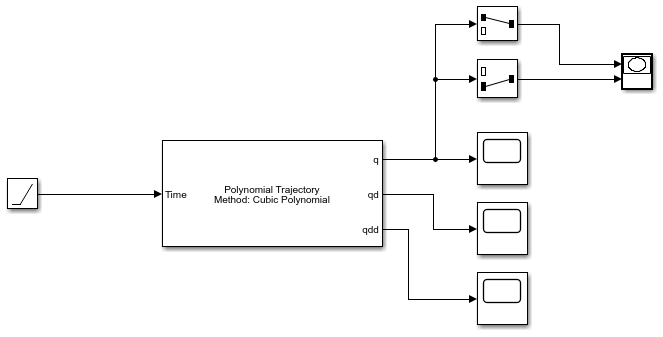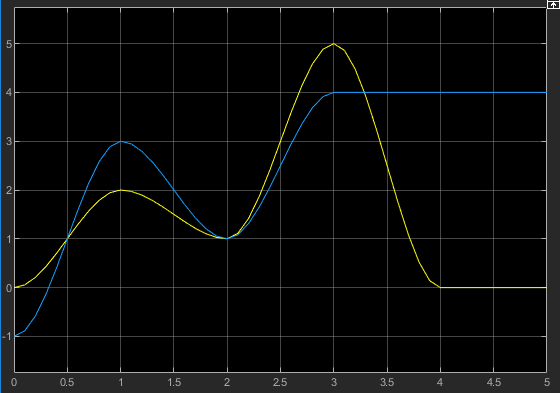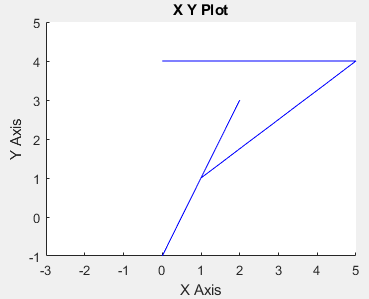# Generate Cubic Polynomial Trajectory

This example shows how to generate a cubic polynomial trajectory using the Polynomial Trajectory block.

Open the model. The block has a set of 2-D waypoints defined in the block mask. The Time input is just a ramp signal to simulate time progressing.

`open_system('cubic_polytraj_ex1.slx')`Run the simulation. The first figure shows the output of the `q` vector for the positions of the trajectory. Notice the cubic polynomial shape to the trajectory between waypoints. The XY Plot shows the actual 2-D trajectory, which hits the waypoints specified in the block mask.